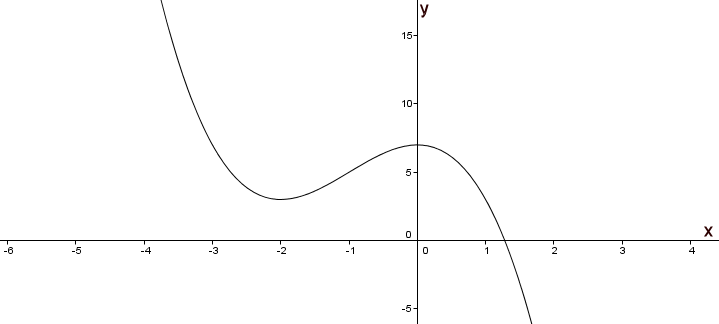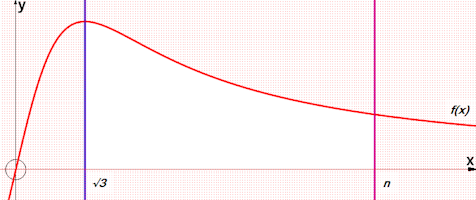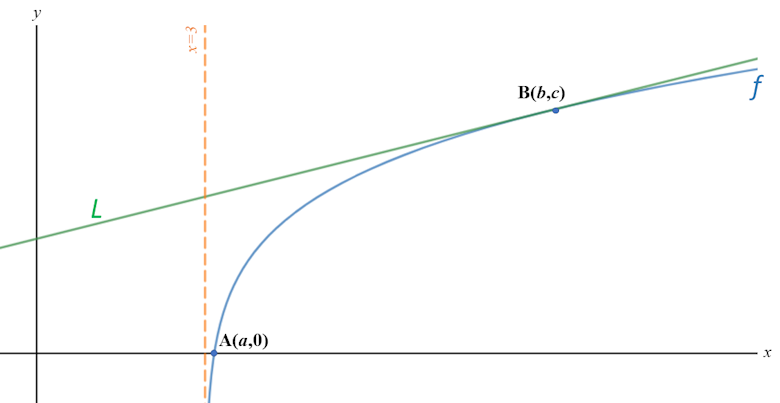# Exam-Style Questions.

## Problems adapted from questions set for previous Mathematics exams.

### 1.

IB Studies

A function is given as $$f(x)=3x^2-6x+4+\frac3x,-2\le x \le 4, x\ne 0$$.

(a) Find the derivative of the function.

(b) Find the coordinates of the local minimum point of $$f(x)$$ in the given domain using your calculator.

### 2.

IB Studies

Consider the graph of the function $$f(x)=7-3x^2-x^3$$(a) Label the local maximum as A on the graph.

(b) Label the local minimum as B on the graph.

(c) Write down the interval where $$f(x)>5$$.

(d) Draw the tangent to the curve at $$x=-3$$ on the graph.

(e) Write down the equation of the tangent at $$x=-3$$.

### 3.

IB Studies

A package is in the shape of a cuboid and has a length $$l$$ cm, width $$w$$ cm and height of 12 cm.

(a) Express the volume of the package in terms of $$l$$ and $$w$$.

The total volume of the package is 2400 cm3.

(b) Show that $$l=\frac{200}{w}$$.

The package is tied up using a length of red string that fits exactly around the package in two different directions, as shown in the following diagram (not to scale).(c) Show that the length of string, $$x$$cm, required to tie up the package can be written as $$24+4w+\frac{400}{w}$$

(d) Sketch the graph of $$x$$ for $$0\lt w \le 12$$, clearly showing the local minimum point.

(e) Find $$\frac{dx}{dw}$$.

(f) Find the value of $$w$$ for which $$x$$ is a minimum.

(g) Find the value, $$l$$, of the package for which the length of string is a minimum.

(h) Find the minimum length of string required to tie up the package.

### 4.

IB Standard

Let $$f(x)=\frac{2x}{x^2+3}$$

(a) Use the quotient rule to show that $$f'(x)=\frac{6-2x^2}{(x^2+3)^2}$$.

(b) Find $$\int \frac{2x}{x^2+3}dx$$.(c) The area enclosed by the graph of $$f(x)$$, the x-axis and the lines $$x=\sqrt3$$ and $$x=n$$ has an area of $$\ln14$$. Find the value of $$n$$.

### 5.

IB Studies

Consider the function $$f(x)=6 - ax+\frac 3{x^2},x\neq 0$$

(a) Write down the equation of the vertical asymptote of the graph of $$y=f(x)$$.

(b) Write down $$f'(x)$$

The line T is the tangent to the graph of $$y=f(x)$$ at the point where $$x=1$$ and it has a gradient of -8.

(c) Show that $$a=2$$.

(d) Find the equation of T.

(e) Using your calculator find the coordinates of the point where the graph of $$y=f(x)$$ intersects the x-axis.

(f) The line T also intersects $$f(x)$$ when $$-2\le x\le 0$$. Find the coordinates of this intersection.

### 6.

IB Analysis and Approaches

Consider the function $$f$$ defined by $$f(x)= \ln{(x^2 - 9)}$$ for $$x > 3$$.

The following diagram shows part of the graph of $$f$$ which crosses the x-axis at point $$A$$ with coordinates $$(a,0)$$.

The line $$L$$ is the tangent to the graph of $$f$$ at the point $$B$$ with coordinates $$(b,c)$$. The gradient of $$L$$ is $$\frac14$$(a) Find the exact value of $$a$$.

(b) Find the value of $$b$$.

### 7.

IB Standard

If $$f(x)=x\sin{x}$$, for $$-3\le x\le3$$

(a) Find $$f'(x)$$

(b) Sketch the graph of $$y=f'(x)$$, for $$-3\le x\le3$$

### 8.

IB Analysis and Approaches

Consider the cubic function $$f(x)=\frac{1}{6}x^3-2x^2+6x-2$$

(a) Find $$f'(x)$$

The graph of $$f$$ has horizontal tangents at the points where $$x = a$$ and $$x = b$$ where $$a < b$$.

(b) Find the value of $$a$$ and the value of $$b$$

(c) Sketch the graph of $$y = f'(x)$$.

(d) Hence explain why the graph of $$f$$ has a local maximum point at $$x = a$$.

(e) Find $$f''(b)$$.

(f) Hence, use your answer to part (e) to show that the graph of $$f$$ has a local minimum point at $$x = b$$.

(g) Find the coordinates of the point where the normal to the graph of $$f$$ at $$x = a$$ and the tangent to the graph of $$f$$ at $$x = b$$ intersect.

### 9.

IB Studies

Consider the function $$f(x)=x^3-9x+2$$.

(a) Sketch the graph of $$y=f(x)$$ for $$-4\le x\le 4$$ and $$-14\le y\le 14$$ showing clearly the axes intercepts and local maximum and minimum points. Use a scale of 2 cm to represent 1 unit on the x-axis, and a scale of 1 cm to represent 2 units on the y-axis.

(b) Find the value of $$f(-1)$$.

(c) Write down the coordinates of the y-intercept of the graph of $$f(x)$$.

(d) Find $$f'(x)$$.

(e) Find $$f'(-1)$$

(f) Explain what $$f'(-1)$$ represents.

(g) Find the equation of the tangent to the graph of $$f(x)$$ at the point where x is –1.

R and S are points on the curve such that the tangents to the curve at these points are horizontal. The x-coordinate of R is $$a$$ , and the x-coordinate of S is $$b$$ , $$b \gt a$$.

(h) Write down the value of $$a$$ ;

(i) Write down the value of $$b$$.

(j) Describe the behaviour of $$f(x)$$ for $$a \lt x \lt b$$.

### 10.

IB Analysis and Approaches

The displacement, in millimetres, of a particle from an origin, O, at time t seconds, is given by $$s(t) = t^3 cos t + 5t sin t$$ where $$0 \le t \le 5$$ .

(a) Find the maximum distance of the particle from O.

(b) Find the acceleration of the particle at the instant it first changes direction.

### 11.

IB Standard

The function $$f$$ is defined as follows:

$$f(x)=\frac{122}{1+60e^{-0.3x}}$$

(a) Calculate $$f(0)$$.

(b) Find a value of $$x$$ for which $$f(x)=85$$

(c) Find the range of $$f$$.

(d) Show that:

$$f'(x)=\frac{2196e^{-0.3x}}{(1+60e^{-0.3x})^2}$$

(e) Find the maximum rate of change of $$f$$.

### 12.

IB Analysis and Approaches

Consider the functions

$$f(x) = m-x^2 - 2xn - n^2$$ $$g(x) = \frac{e^x}{e^2} + \frac{m}{2}$$

where $$m, n \in \mathbb R$$

(a) Find $$f'(x)$$.

(b) Find $$g'(x)$$.

The graphs of $$f$$ and $$g$$ have a common, non-vertical tangent at $$x=3$$.

(c) Find expressions for $$m$$ and $$n$$ in terms of $$e$$.

### 13.

IB Standard

Let $$f(x)=jx^3+jx^2+kx+m$$ where $$j, k$$ and $$m$$ are constants.

(a) Find $$f'(x)$$

(b) If $$f'(x)\ge 0$$, show that $$j^2\le 3jk$$.

### 14.

IB Standard

Make a sketch of a graph showing the velocity (in $$ms^{-1}$$) against time of a particle travelling for six seconds according to the equation:

$$v=e^{\sin t}-1$$

(a) Find the point at which the graph crosses the $$t$$ axis.

(b) How far does the particle travel during these first six seconds?

### 15.

IB Analysis and Approaches

The function $$f$$ is such that $$f(x) = \frac{\ln2x}{x^3}$$ where $$x \gt 0$$.

(a) Find the first derivative of the function, $$f'(x)$$.

(b) The graph of $$y=f(x)$$ has a horizontal tangent at the point T. Find the coordinates of T.

(c) Show that T is a local maximum point by considering the second derivative, $$f''(x)$$.

(d) Find the values of $$x$$ for which $$f(x) \gt 0$$.

(e) Sketch the graph of $$f$$ showing clearly the value of the x-intercept and the approximate position of point T.

### 16.

IB Analysis and Approaches

Consider the function $$f$$ defined by $$f(x) = 25e^{x-5}$$ for $$x \in \mathbb{R}^+$$.

(a) Find the coordinates of the points where the graph of $$f$$ intersects the line $$y=x$$.

The line $$L$$ has a gradient of $$-1$$ and is a normal to the graph of $$f$$ at the point $$R$$.

(b) Find the exact coordinates of $$R$$.

(c) Show that the equation of the line $$L$$ is $$y=-x+6- \ln{25}$$.

(d) Find the area of the region enclosed by the graph of $$f$$ and its inverse.

### 17.

IB Analysis and Approaches

Let $$f(x) = \frac{ln3x}{kx}$$ where $$x \gt 0$$ and $$k \in \mathbf Q^+$$.

(a) Find an expression for the first derivative $$f'(x)$$.

The graph of $$f$$ has exactly one maximum point at P.

(b) Find the x-coordinate of P.

The graph of $$f$$ has exactly one point of inflection at Q.

(c) Find the x-coordinate of Q.

(d) The region enclosed by the graph of $$f$$, the x-axis, and the vertical lines through P and Q has an area of one square unit, find the value of $$k$$.

### 18.

IB Studies

Consider the function $$f(x)=\frac{20}{x^2}+kx$$ where $$k$$ is a constant and $$x\neq0$$.

(a) Write down $$f'(x)$$

The graph of $$y = f(x)$$ has a local minimum point at $$x=2$$.

(b) Show that $$k=5$$.

(c) Find $$f(1)$$.

(d) Find $$f'(1)$$.

(e) Find the equation of the normal to the graph of $$y=f(x)$$ at the point where $$x=1$$
Give your answer in the form $$ay+bx+c=0$$ where $$a, b, c \in \mathbb{Z}$$

(f) Sketch the graph of $$y=f(x)$$ , for $$-5\le x\le 10$$ and $$-10\le y\le 50$$.

(g) Write down the coordinates of the point where the graph of $$y=f(x)$$ intersects the x-axis.

(h) State the values of $$x$$ for which $$f(x)$$ is decreasing.

If you would like space on the right of the question to write out the solution try this Thinning Feature. It will collapse the text into the left half of your screen but large diagrams will remain unchanged.

The exam-style questions appearing on this site are based on those set in previous examinations (or sample assessment papers for future examinations) by the major examination boards. The wording, diagrams and figures used in these questions have been changed from the originals so that students can have fresh, relevant problem solving practice even if they have previously worked through the related exam paper.

The solutions to the questions on this website are only available to those who have a Transum Subscription.

Exam-Style Questions Main Page

Search for exam-style questions containing a particular word or phrase:

To search the entire Transum website use the search box in the grey area below.